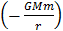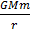# A satellite is moving with a constant speed v in circular orbit around the earth. An object of mass ‘m’ is ejected from the satellite such that it just escapes from the gravitational pull of earth. At the time of  ejection, the kinetic energy of the object is : (a)(b)(c) 2(d)## Question ID - 50220 :- A satellite is moving with a constant speed v in circular orbit around the earth. An object of mass ‘m’ is ejected from the satellite such that it just escapes from the gravitational pull of earth. At the time of  ejection, the kinetic energy of the object is : (a)(b)(c) 2(d)3537

At height r from center of earth. Orbital velocity

=By  energy conservation

KE of ‘m’ +=0+0

(At infinity, PE=KE=0)

⇒KE of ‘m’==m=Next Question :
 A solid metal cube of edge length 2 cm is moving in a positive y direction at a constant speed of 6m/s. there is a uniform magnetic field of 0.1 T in the positive z-direction. The potential difference between the two faces of the cube perpendicular to the x-axis, is: (a) 6 mV (b) 1 mV (c) 12 mV (d) 2 mV